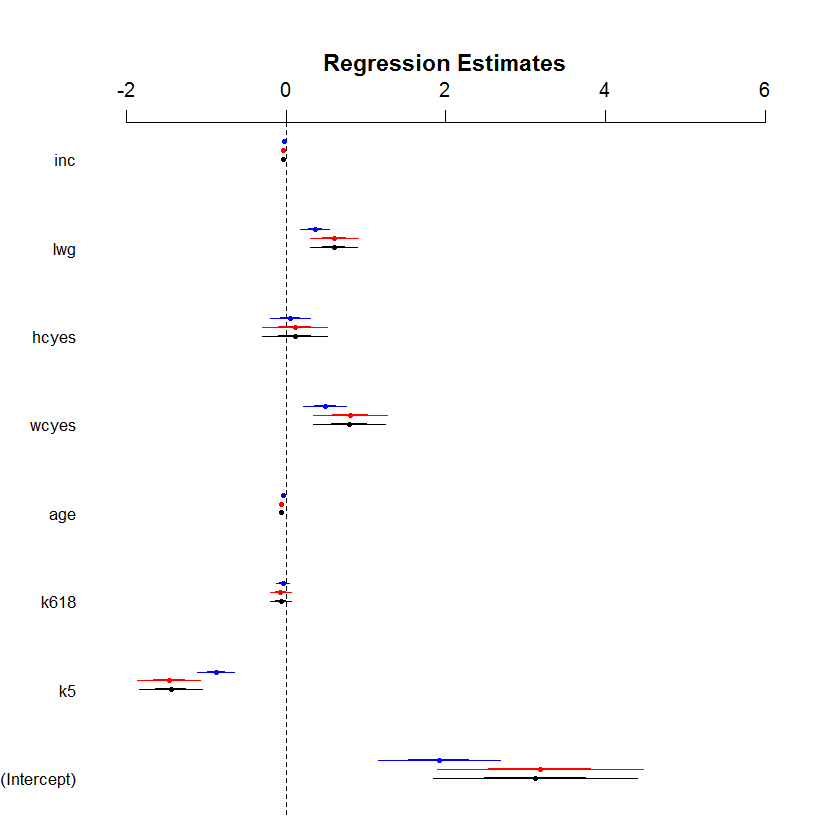# Visualization of regression coefficients (in R)

July 2, 2010
By

Want to share your content on R-bloggers? click here if you have a blog, or here if you don't.

Update (07.07.10): The function in this post has a more mature version in the “arm” package. See at the end of this post for more details.
* * * *

Imagine you want to give a presentation or report of your latest findings running some sort of regression analysis. How would you do it?

This was exactly the question Wincent Rong-gui HUANG has recently asked on the R mailing list.

One person, Bernd Weiss, responded by linking to the chapter “Plotting Regression Coefficients” on an interesting online book (I have never heard of before) called “Using Graphs Instead of Tables” (I should add this link to the free statistics e-books list…)

Letter in the conversation, Achim Zeileis, has surprised us (well, me) saying the following

I’ve thought about adding a plot() method for the coeftest() function in the “lmtest” package. Essentially, it relies on a coef() and a vcov() method being available – and that a central limit theorem holds. For releasing it as a general function in the package the code is still too raw, but maybe it’s useful for someone on the list. Hence, I’ve included it below.

(I allowed myself to add some bolds in the text)

So for the convenience of all of us, I uploaded Achim’s code in a file for easy access. Here is an example of how to use it:

``` source("http://www.r-statistics.com/wp-content/uploads/2010/07/coefplot.r.txt")   data("Mroz", package = "car") fm <- glm(lfp ~ ., data = Mroz, family = binomial) coefplot(fm, parm = -1) ```

I hope Achim will get around to improve the function so he might think it worthy of joining his“lmtest” package. I am glad he shared his code for the rest of us to have something to work with in the meantime* * *

Update (07.07.10):
Thanks to a comment by David Atkins, I found out there is a more mature version of this function (called coefplot) inside the {arm} package. This version offers many features, one of which is the ability to easily stack several confidence intervals one on top of the other.

It works for baysglm, glm, lm, polr objects and a default method is available which takes pre-computed coefficients and associated standard errors from any suitable model.

Example:
(Notice that the Poisson model in comparison with the binomial models does not make much sense, but is enough to illustrate the use of the function)

``` library("arm") data("Mroz", package = "car") M1<- glm(lfp ~ ., data = Mroz, family = binomial) M2<- bayesglm(lfp ~ ., data = Mroz, family = binomial) M3<- glm(lfp ~ ., data = Mroz, family = binomial(probit)) coefplot(M2, xlim=c(-2, 6), intercept=TRUE) coefplot(M1, add=TRUE, col.pts="red", intercept=TRUE) coefplot(M3, add=TRUE, col.pts="blue", intercept=TRUE, offset=0.2) ```

(hat tip goes to Allan Engelhardt for help improving the code, and for Achim Zeileis in extending and improving the narration for the example)

Resulting plot* * *
Lastly, another method worth mentioning is the Nomogram, implemented by Frank Harrell’a rms package.

R-bloggers.com offers daily e-mail updates about R news and tutorials about learning R and many other topics. Click here if you're looking to post or find an R/data-science job.
Want to share your content on R-bloggers? click here if you have a blog, or here if you don't.

Tags: , , , , , , , , ,